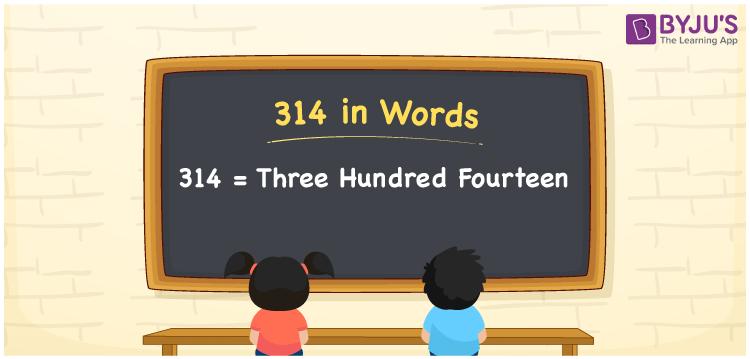# 314 in Words

314 in words is Three hundred fourteen. It is known that we can write the word form of single and double-digit numbers quickly, but to get the word form of three and more digit numerals, we must utilise the place value chart. Word forms of numbers are used to spell or communicate the numerals accurately. For instance, Rs. 314 can be spelt as Three hundred fourteen rupees. Here, 314 is a cardinal number since it represents a specific amount.

 314 in words Three hundred fourteen Three hundred fourteen in Numbers 314

## 314 in English Words

We generally write numbers in words using the English alphabet. Thus, we spell 314 in English words as “Three hundred fourteen”.## How to Write 314 in Words?

The conversion of the number 314 to word form is simple when we use the place value chart for 314, which is illustrated below.

 Hundreds Tens Ones 3 1 4

Here, ones = 4, tens = 1, hundreds = 3

Thus, by expanding these numbers, we get;

3 × Hundred + 1 × Ten + 4 × One

= 3 × 100 + 1 × 10 + 4 × 1

= 300 + 10 + 4

= Three hundred + Fourteen {since ten + four = fourteen}

= Three hundred fourteen

Therefore, 314 in words = Three hundred fourteen.

314 is a natural number that precedes 315 and succeeds 313.

314 in words – Three hundred fourteen

Is 314 an even number? – Yes

Is 314 an odd number? – No

Is 314 a prime number? – No

Is 314 a composite number? – Yes

Is 314 a perfect square number? – No

Is 314 a perfect cube number? – No

## Frequently Asked Questions on 314 in Words

Q1

### How do you write 314 in words?

The numeral 314 can be written in words as Three hundred fourteen.
Q2

### How to write Rs. 314 in words on a cheque?

On a cheque, we generally write an amount of Rs. 314 in words as “Three hundred fourteen rupees only”.
Q3

### Express the number 314.75 in words.

The decimal number 314.75 can be expressed in words as “Three hundred fourteen point seven five”.